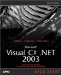# Using Late Binding

Late binding is the process of waiting until runtime to bind a method to an object. With late binding, you don't have to specify what object you're going to work with until the code is actually running. This is a useful skill because you can create an instance of an assembly (such as Microsoft Access or Excel) and invoke its methods using late binding.

Here's an example. Say you have a class named Calculator with a method named Addem that adds two integers:

` `
` public class Calculator {   public virtual long Addem(int x, int y)   {     return x + y;   } } `

Now say you want to invoke the Addem method at runtime using late binding. We'll start by creating a new instance of the Calculator type using the Activator class's CreateInstance method. We could just create a Calculator object in code with new , of course, but we're demonstrating the CreateInstance method here because you can use this method to create objects from external assemblies and other remote objects:

` `
` Type calculatorType = Type.GetType("Calculator"); Object calculator = Activator.CreateInstance(calculatorType); `

In fact, the Activator class specializes in creating objects from assemblies and other external objects; here are its static members , which you use for this purpose:

• CreateComInstanceFrom Creates an instance of a COM object.

• CreateInstance Creates an instance of the specified type.

• CreateInstanceFrom Creates an instance using an assembly file.

• GetObject Creates a proxy for a currently running remote object, server-activated object, or Web service.

To call the Addem method of the new calculator object, we need to indicate which parameters this method takes, which we can do by creating a Type array:

` `
` Type[] parameterTypes = new Type; parameterTypes= Type.GetType("System.Int32"); parameterTypes= Type.GetType("System.Int32"); `

We also need to create a MethodInfo object holding information about the method we're about to call. You're free to specify the name of the method at runtime, which is what late binding is all about:

` `
` Type type = Type.GetType("Calculator"); MethodInfo methodInfo = type.GetMethod("Addem", parameterTypes); `

And we need to set up an array with the data we want to pass to this method; in this case, we'll add 2 and 3:

` `
` Object[] parameters = new Object; parameters = 2; parameters = 3; `

Finally, you can call the Invoke method of the MethodInfo object to invoke the Addem method, as you see in ch14_07.cs, Listing 14.7.

##### Listing 14.7 Late Binding (ch14_07.cs)
` using System; using System.Reflection; public class Calculator {   public virtual long Addem(int x, int y)   {     return x + y;   } } class ch14_07 {   public static void Main()   {    Type calculatorType = Type.GetType("Calculator");    Object calculator = Activator.CreateInstance(calculatorType);    Type[] parameterTypes = new Type;    parameterTypes= Type.GetType("System.Int32");    parameterTypes= Type.GetType("System.Int32");    Type type = Type.GetType("Calculator");    MethodInfo methodInfo = type.GetMethod("Addem", parameterTypes);    Object[] parameters = new Object;    parameters = 2;    parameters = 3;  System.Console.WriteLine("Addem(2, 3) = {0}",   methodInfo.Invoke(calculator, parameters));  } } `

Here are the results you see when you run ch14_07:

` `
` C:\>ch14_07 Addem(2, 3) = 5 `

As you can see, we've been able to late-bind a method, specifying which method of an object to bind at runtime, not at compile time. In this way, late binding lets you customize your code at runtime. In fact, the fourth reflection technique, reflection emit, lets you customize your code entirely at runtime, by writing it from scratch.Microsoft Visual C#.NET 2003 Kick Start
ISBN: 0672325470
EAN: 2147483647
Year: 2002
Pages: 181

Similar book on Amazon